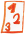Math worksheetsCustom math worksheets at your fingertips

# Details for problem "Extract binomic formula from term"

Quickname: 1825

## Summary

A binomic formula is hidden in a term. Factor out the binomic formular or extract the summand.

## Example## Description

The expanded term for a binomic formula is part of a given term. The given term has been created by multiplying the binomic formular with a factor or by adding a summand or difference. The task is to extract the binomic formula and convert it to the original form written as a factor of two summands. To perform this, either the factor applied has to be factored out or the additional summand has to be extracted.

The summands of the formula's factors will be either a variable and a literal number or both of them will be variables. In no case, both summands will be numbers, which means the expanded term will always contain variables and it does not simplify to a single literal number.

The number of problems is selectable. For the types of formulas that will appear it can be specified that only some of the three binomic formulas will be used. The modifying term can be either a product, a sum or a difference. There is an option to choose with of these arithmetic operations can appear.

Variables that appear will always be named a and b or x and y.

Download free worksheets for this math problem here. There is a second sheet with the solutions. Just click on the respective link.Worksheet 1Solution sheetWorksheet 2Solution sheetWorksheet 3Solution sheet

If you can not see the solution sheets for download, they may be filtered out by an ad blocker that you may have installed. If this is the case, please allow ads for this page and reload the page. The solution sheets will then reappear.

• Do the sample worksheets do not really fit?
• Do you need more math worksheets, with a different level of difficulty?
• Would you like to combine different problems on a worksheet and adjust them to your needs?
• As a teacher, you can put together your own worksheets using the automatically generated math problems provided.
With a free initial credit, you can start creating your own math worksheets in a few minutes.

It does not cost anything to try! Register here, to create custom worksheets now!

## Customization options for this problem

ParameterPossible values
Number of problems1, 2, 3, 4, 5, 6, 7, 8, 9, 10
Binomic formula type(a+b)^2, (a-b)^2, (a+b)(a-b), (a+b)^2 & (a-b)^2, all
Part ofproduct,sum,difference, product, sum, sum,difference
Other termvariable,number, variable, number
Provide hintYes, No